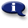# .

## Syllabus Information

Winter 2021
May 18,2022Use this page to maintain syllabus information, learning objectives, required materials, and technical requirements for the course.

Syllabus Information
MTH 095 - Intermediate Algebra
Associated Term: Winter 2021
Learning Objectives: Upon successful completion of this course, the student should be able to: 1. Use prerequisite concepts and skills of the arithmetic of real numbers. 2. Manipulate and evaluate expressions involving exponents, and use scientific notation. 3. Simplify expressions involving polynomials, including factoring. 4. Simplify and perform operations involving rational expressions. 5. Solve equations involving rational expressions. 6. Solve application problems leading to equations involving rational expressions. 7. Use function notation and distinguish between input and output. 8. Write the equation of a line and graph lines on a rectangular coordinate system. 9. Solve linear equations and apply linear equations to application problems. 10. Solve and graph a linear inequality, and use interval notation. 11. Simplify and perform operations involving radical expressions. 12. Solve equations involving radical expressions. 13. Solve application problems leading to equations involving rational expressions. 14. Write the square root of a negative number in terms of i, and operate with complex numbers. 15. Solve quadratic equations by taking square roots, by completing the square, and by the quadratic formula. 16. Given a quadratic function find its vertex, axis of symmetry, and intercepts, and graph the parabola. 17. Model and solve application problems involving quadratic equations and functions. 18. Evaluate an exponential function. 19. Translate between equivalent exponential and logarithmic notations. 20. Model and solve application problems involving exponential and logarithmic equations. 21. Use a scientific calculator when appropriate and in an efficient manner.
Required Materials:
Technical Requirements: